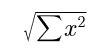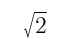# Mission Statement of MDSM Research Foundation

Mission Statement of MDSM Research Foundation

ABSTRACT:

MDSM has been developed for system trend analysis. The model was originated from diagonal matrix. Most of the research papers were published in a monograph, titled “趋势分析及其在生态股市中的应用 (Trend Analysis and Its Applications on Ecology and Stock Market)”, by The Ethnic Publishing House, Beijing, 2006. The Foundation is to find resources to translate and publish the book in the USA

In order to promote, publish, and popularize the MDSM research, a foundation was established.

MDSM is an abbreviation for the Multi-Dimensional -Sphere Model.

T. Jay Bai has developed the MDSM for prediction of Rangeland Degradation (1984) and Land Condition Trend Analysis (1997). This math model is originated from Diagonal Matrix (1984).

Instead of using real number in one dimensional space, the MDSM uses multicomponent vectors (m-Vectors) in multi-dimensional space to express a multivariate-systems, as we consider the world is made of systems, instead of variables.

According to Grolier Encyclopedia (1999), the m-Vectors are the variables with both Magnitude and Directions.

The Magnitude of an m-Vectors is expressed by their Square root of the Sum of the Squares,

|m-Vector|=SSS=Thus, it stands in multidimensional space, that |1|+|1|=||, or 1+1=. This new definition of addition will lead to a new branch of Algebra, i.e. Vector Algebra, or Hyper Algebra.

The directions of the Vectors are expressed by their cosine values, or the standardized Vectors: cosine=vector/|vector|. Thus, the directions are used to express the states of an m-Systems.

A component-wise multiplication and division has been defined by MDSM (1997). So that the model can use quotient vectors to express the “state transition trends”:

Tk=k/(k-1), when T>1, means increasing, T<1, means decreasing

Furthermore, the MDSM can use the second order trends, TT, to express the turning point of the systems:

TTk=Tk:T(k-1) =k/k-1:(k-1/k-2=k*(k-2):(k-1)2, when TT>1, means concave, TT<1, means convex,

(Where k, k-1, and k-2 are “present”, “previous”, and “pre-previous” states of the systems).

These researches were applied in different fields and proven to be working, and repeatable.

Most of the research papers are collected in Jay’s monograph, which was published in 2006 by The Ethnic Publishing House, Beijing, in Chinese. The title of the book is 趋势分析及其在生态股市中的应用 (Trend Analysis and Its Applications on Ecology and Stock Market).

As the MDSM is new math and could be a powerful weapon, this research eventually should belong to all people, instead of any private company. The purpose of the MDSM Research Foundation is to find financial resources and human resources to help us to translate and publish the book in the United States in English. We will start with people by presenting series seminars.

https://m.sciencenet.cn/blog-333331-1195354.html

## 全部精选博文导读

GMT+8, 2023-6-1 06:50